FreeRDP
TestFreeRDPRegion.c File Reference
`#include <winpr/crt.h>`
`#include <winpr/print.h>`
`#include <freerdp/codec/region.h>`

## Typedefs

typedef int(* TestFunction) (void)

## Functions

static BOOL compareRectangles (const RECTANGLE_16 *src1, const RECTANGLE_16 *src2, int nb)

static int test_basic (void)

static int test_r1_r3 (void)

static int test_r9_r10 (void)

static int test_r1_r5 (void)

static int test_r1_r6 (void)

static int test_r1_r2_r4 (void)

static int test_r1_r7_r8 (void)

static int test_r1_r2_r3_r4 (void)

static int test_from_weston (void)

static int test_r1_inter_r3 (void)

static int test_r1_r3_inter_r11 (void)

static int test_norbert_case (void)

static int test_norbert2_case (void)

static int test_empty_rectangle (void)

int TestFreeRDPRegion (int argc, char *argv[])

## Variables

static struct UnitaryTest tests []

## ◆ TestFunction

 typedef int(* TestFunction) (void)

## ◆ compareRectangles()

 static BOOL compareRectangles ( const RECTANGLE_16 * src1, const RECTANGLE_16 * src2, int nb )
static

FreeRDP: A Remote Desktop Protocol Implementation

Licensed under the Apache License, Version 2.0 (the "License"); you may not use this file except in compliance with the License. You may obtain a copy of the License at

Unless required by applicable law or agreed to in writing, software distributed under the License is distributed on an "AS IS" BASIS, WITHOUT WARRANTIES OR CONDITIONS OF ANY KIND, either express or implied. See the License for the specific language governing permissions and limitations under the License.

Here is the caller graph for this function: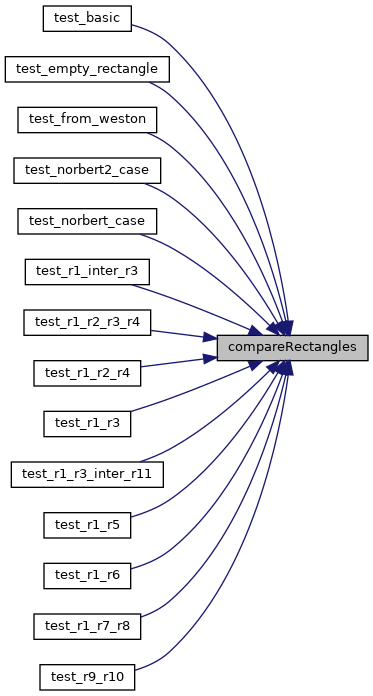## ◆ test_basic()

 static int test_basic ( void )
static
Here is the call graph for this function: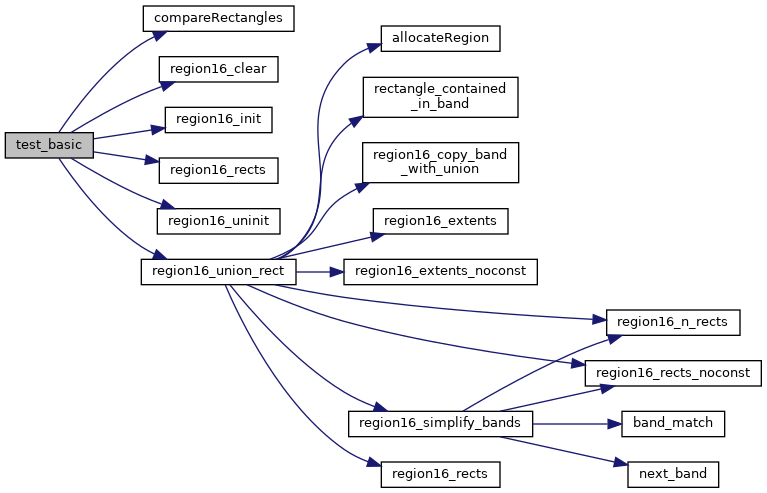## ◆ test_empty_rectangle()

 static int test_empty_rectangle ( void )
static
Here is the call graph for this function:## ◆ test_from_weston()

 static int test_from_weston ( void )
static
Here is the call graph for this function: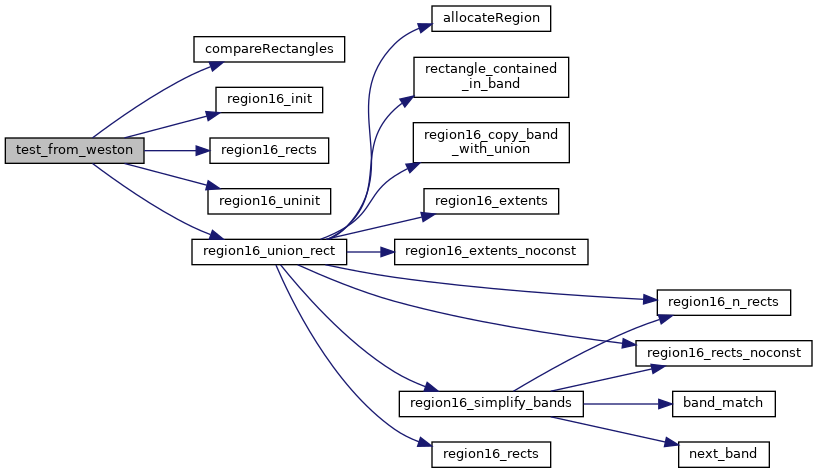## ◆ test_norbert2_case()

 static int test_norbert2_case ( void )
static
Here is the call graph for this function:## ◆ test_norbert_case()

 static int test_norbert_case ( void )
static
Here is the call graph for this function: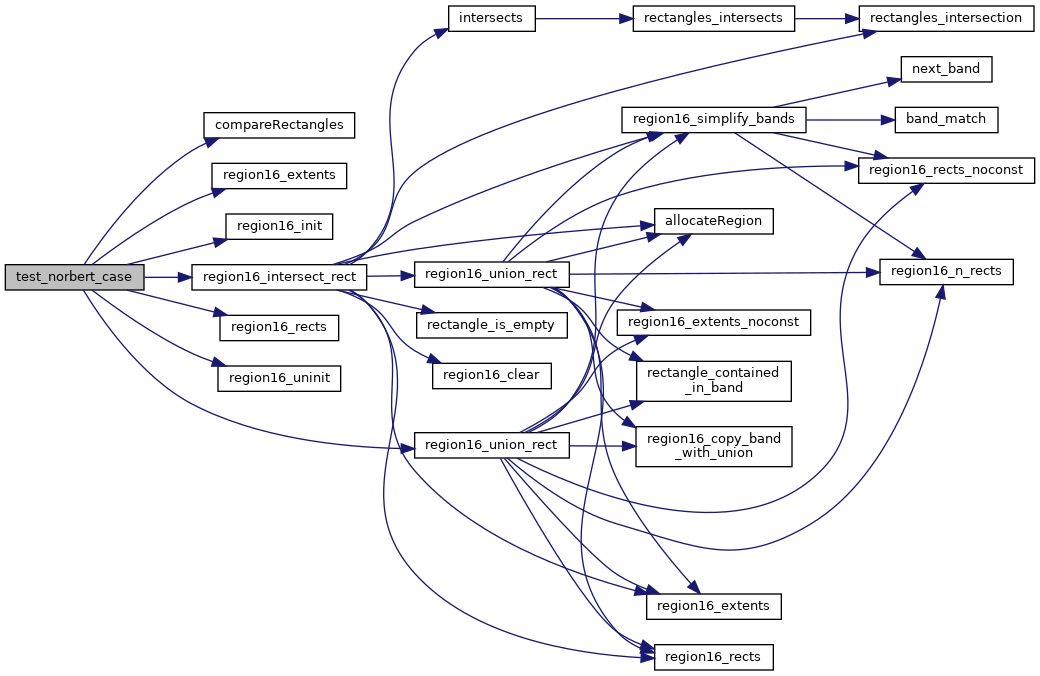## ◆ test_r1_inter_r3()

 static int test_r1_inter_r3 ( void )
static
Here is the call graph for this function: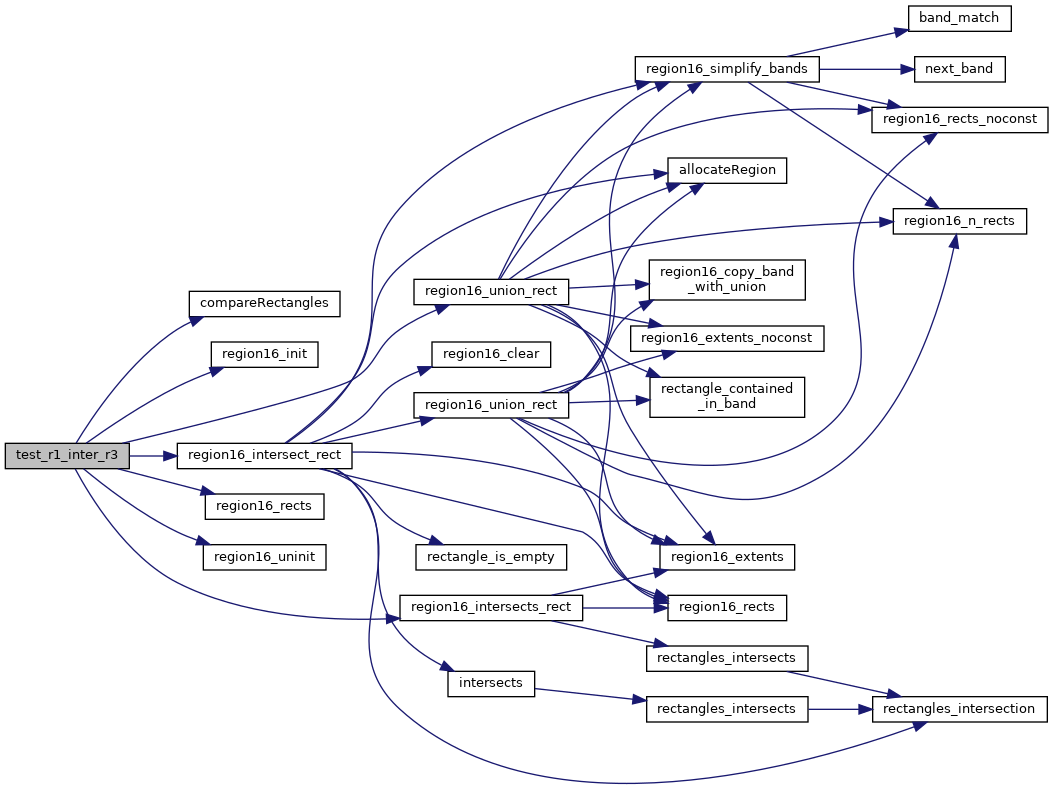## ◆ test_r1_r2_r3_r4()

 static int test_r1_r2_r3_r4 ( void )
static
Here is the call graph for this function:## ◆ test_r1_r2_r4()

 static int test_r1_r2_r4 ( void )
static
Here is the call graph for this function: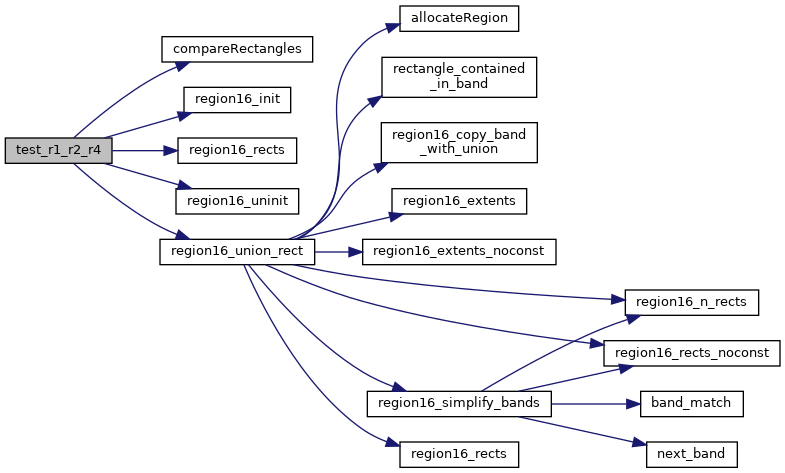## ◆ test_r1_r3()

 static int test_r1_r3 ( void )
static
Here is the call graph for this function: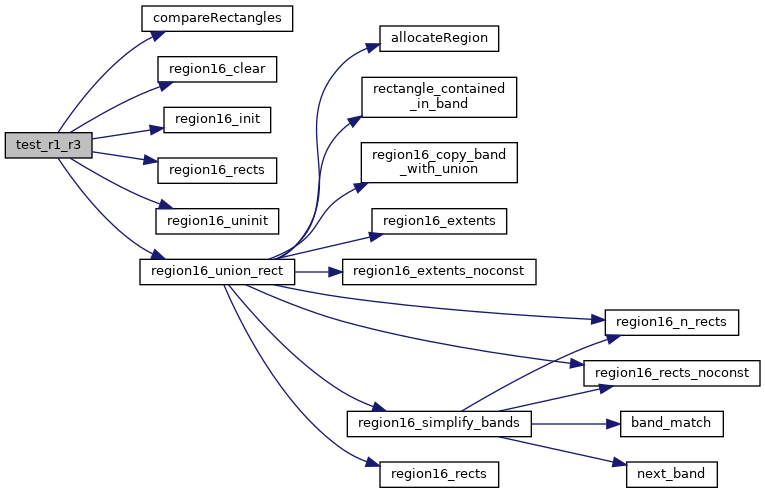## ◆ test_r1_r3_inter_r11()

 static int test_r1_r3_inter_r11 ( void )
static
Here is the call graph for this function: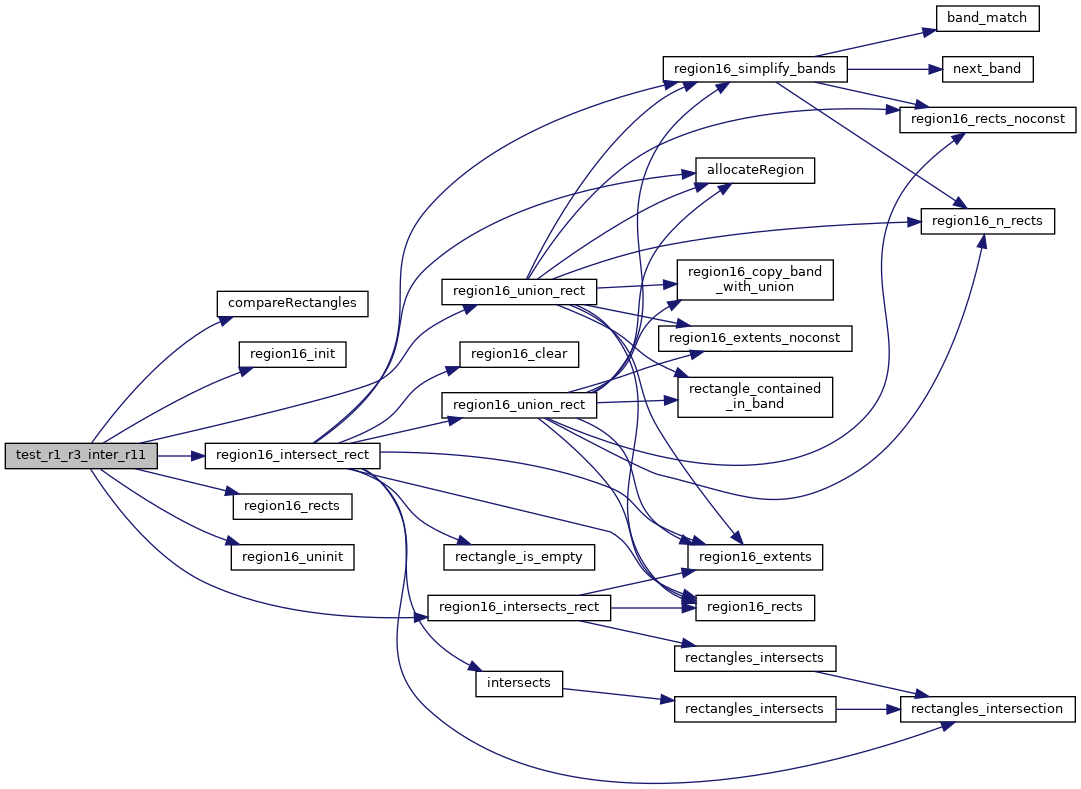## ◆ test_r1_r5()

 static int test_r1_r5 ( void )
static
Here is the call graph for this function: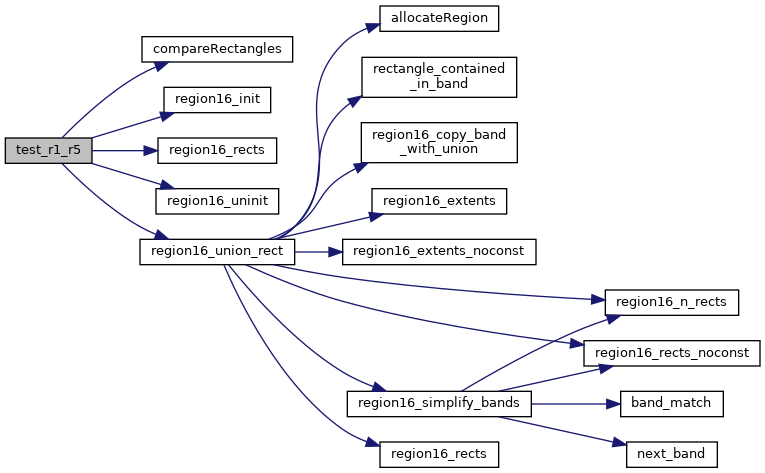## ◆ test_r1_r6()

 static int test_r1_r6 ( void )
static
Here is the call graph for this function: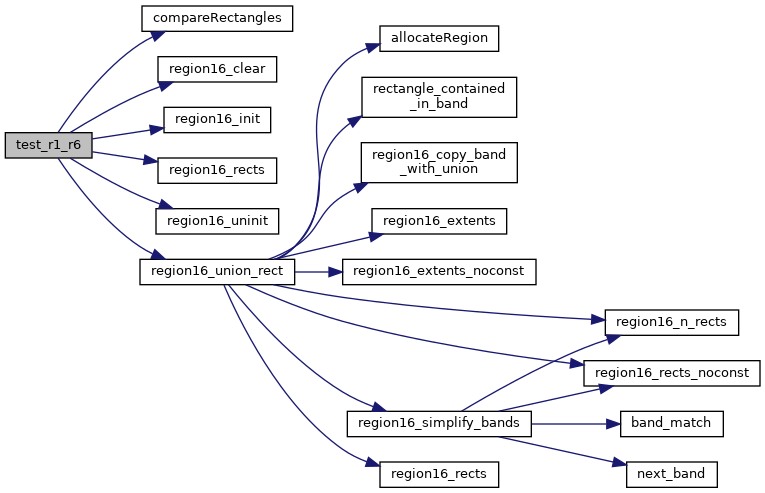## ◆ test_r1_r7_r8()

 static int test_r1_r7_r8 ( void )
static
Here is the call graph for this function:## ◆ test_r9_r10()

 static int test_r9_r10 ( void )
static
Here is the call graph for this function:## ◆ TestFreeRDPRegion()

 int TestFreeRDPRegion ( int argc, char * argv[] )

## ◆ tests

 struct UnitaryTest tests[]
static
Initial value:
= { { "Basic trivial tests", test_basic },
{ "R1+R3 and R3+R1", test_r1_r3 },
{ "R1+R5", test_r1_r5 },
{ "R1+R6", test_r1_r6 },
{ "R9+R10", test_r9_r10 },
{ "R1+R2+R4", test_r1_r2_r4 },
{ "R1+R7+R8 in many orders", test_r1_r7_r8 },
{ "R1+R2+R3+R4", test_r1_r2_r3_r4 },
{ "data from weston", test_from_weston },
{ "R1 & R3", test_r1_inter_r3 },
{ "(R1+R3)&R11 (band merge)", test_r1_r3_inter_r11 },
{ "norbert's case", test_norbert_case },
{ "norbert's case 2", test_norbert2_case },
{ "empty rectangle case", test_empty_rectangle },
{ NULL, NULL } }
test_r1_r5
static int test_r1_r5(void)
Definition: TestFreeRDPRegion.c:187
test_r1_r3_inter_r11
static int test_r1_r3_inter_r11(void)
Definition: TestFreeRDPRegion.c:571
test_empty_rectangle
static int test_empty_rectangle(void)
Definition: TestFreeRDPRegion.c:771
test_norbert2_case
static int test_norbert2_case(void)
Definition: TestFreeRDPRegion.c:699
test_r1_r2_r4
static int test_r1_r2_r4(void)
Definition: TestFreeRDPRegion.c:268
test_r1_r3
static int test_r1_r3(void)
Definition: TestFreeRDPRegion.c:88
test_r1_r6
static int test_r1_r6(void)
Definition: TestFreeRDPRegion.c:228
test_r1_r2_r3_r4
static int test_r1_r2_r3_r4(void)
Definition: TestFreeRDPRegion.c:396
test_r9_r10
static int test_r9_r10(void)
Definition: TestFreeRDPRegion.c:142
test_norbert_case
static int test_norbert_case(void)
Definition: TestFreeRDPRegion.c:630
test_r1_inter_r3
static int test_r1_inter_r3(void)
Definition: TestFreeRDPRegion.c:525
test_basic
static int test_basic(void)
Definition: TestFreeRDPRegion.c:45
test_from_weston
static int test_from_weston(void)
Definition: TestFreeRDPRegion.c:473
NULL
if availableBytes return NULL
Definition: TPCircularBuffer.h:109
test_r1_r7_r8
static int test_r1_r7_r8(void)
Definition: TestFreeRDPRegion.c:317